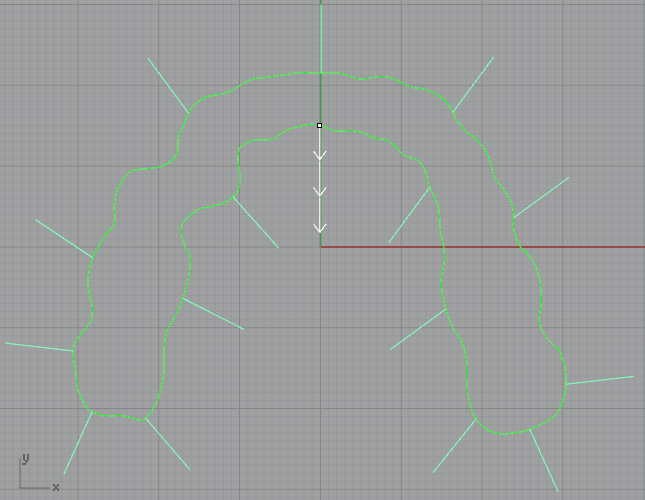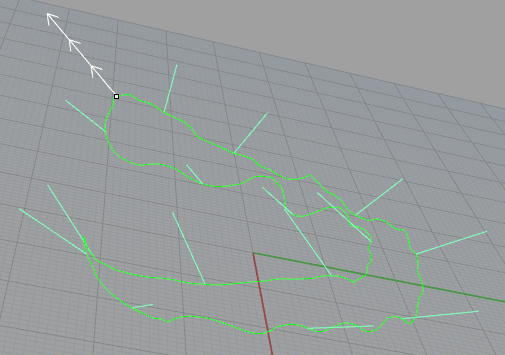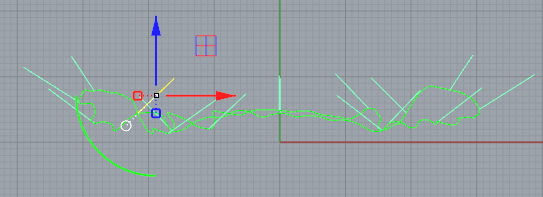# Move the line to the curve with python

Hi everyone)
Set out to automate the routine.
I’ll be honest, in Rnino I just started to get used to it.
Slightly better (than Rino) familiar with Python.

I need help/hints on the following question, please.
Available:
curve (name:trimline)
16 vectors (name:vector_0…15; lines at an angle of 33 degrees on the z-axis).
See:
pic_1

pic_2

I need to write a python script to move the “vectors”, their initial bottom point, to the same position as the point on the “curve”.
The curve is built in the for loop, line-by-line reading from the txt file.
You need to programmatically move these “vectors” (keeping their location in space)
pic_3pic_4pic_5``````import rhinoscriptsyntax as rs
import random

raw_points = [[float(i) for i in s.strip(' \n').split()] for s in open(r'C:\Users\User\Desktop\trimmingline.txt', 'r').readlines()]
clear_points = raw_points[::2]

def create_trimline(points=clear_points):
rs.Command("_SelAll _Lock")

# 1 - Draw curve
for point in range(1, len(points)):

# 2 - Select all curves and joined into one curve
rs.Command("_SelAll _Join")

trimline = rs.SelectedObjects()

# 3 - Checking that the curve is closed
if rs.IsCurveClosed(trimline) and rs.IsCurveClosable(trimline):
print("Trimlines curve created successfully")
else:
rs.SelectObject(trimline)
rs.Command('_CloseCrv')
print("Curve create")
#rs.CloseCurve(trimline) - does not close curve

# - - Set object name and layer
rs.ObjectName(trimline, "trimlines")
rs.ObjectLayer(trimline, "Lines")

# 4 - Smooth trimline
for i in range(5):
rs.Command("_-Smooth SmoothFactor={} CoordinateSystem=World  X=Yes  Y=Yes  Z=Yes  FixBoundaries=Yes _Enter".format(round(random.uniform(0.2, 0.8), 2)))
# in command line: Unknown command smoothfactor and "bla bla bla"
#Rhino.SmoothCurve(trimline, 0.4, True, True, True, True, intCoordinateSystem=1)

# 5 - Control Seam point (Flip=No)
if rs.IsCurveClosed(trimline):
domain = rs.CurveDomain(trimline)
parameter = (domain + 13)
rs.CurveSeam(trimline, parameter)
print("Seam line installed on (Flip=No)")

rs.UnselectAllObjects()
rs.Command("_Unlock")
``````

And if it doesn’t make it difficult for someone who has come back, tell me by the code, in places # 1, 2, 3, 4, 5 … How to improve to simplify.